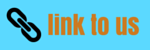# Rotation

## (12) Angular momentum of the system of particles with respect to the center of mass of the system

• let a system of particles is made up of n number of particles.Let ri be the position vector of the ith particle P with respect to a poiint O and vi be its velocity .let Rcm be the position vector of center of mass C of the system with respect to the origin
• Let ri' and vi' be the position vector and velocity vector of the ith particle with respect to center of mass of the system.
• Angular momentum of the system of particles with respect to origin is given by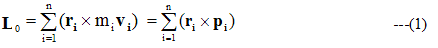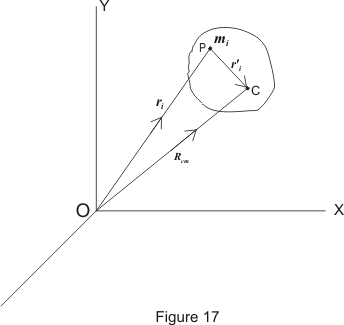• Angular momentum of the system of particles with respect to center of mass of the system is given by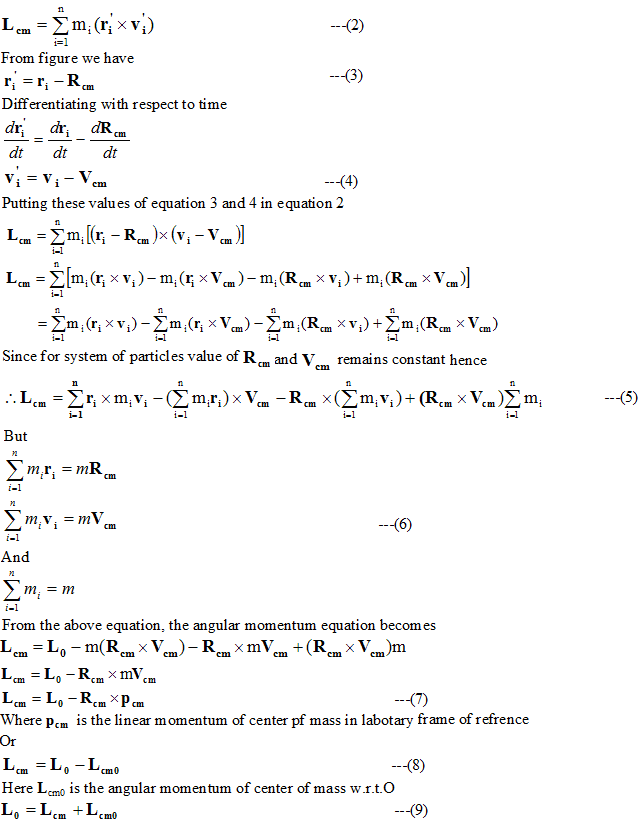Hence the angular momentum of the system of the particles with respect to point O is equal to the sum of the angular momentum of the center of mass of the particles about O and angular monentum of the system about center of mass

(13) Law of conservation of angular momentum
• Torque acting on any particle is given by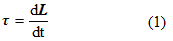If external torque acting on any particle os zero then,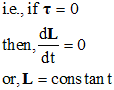• Hence in absence of external torque the angular momentum of the particle remains constant or conserved.
• Total torque acting on any system is given by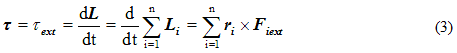• If total external force acting on any particle system is zero or,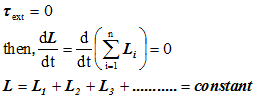• If total external torque acting on any body is zero , then total angular momentum of the body remains constant or conserved.